# Colors

In general, colors for categorial variables are set as `{cell: color}` dictionaries. For factors, cells are strings, and for interactions they are tuples of strings. Colors can be set to any color understood by matplotlib.

```# sphinx_gallery_thumbnail_number = 3
from eelbrain import *
import matplotlib.style

ds = datasets.get_uv()

colors = {'a1': (1, 0, 0), 'a2': (0, 0, 1)}
p = plot.Barplot('fltvar', 'A', data=ds, w=2, colors=colors)

colors = {
('a1', 'b1'): 'red',
('a1', 'b2'): (1, 1, 0),
('a2', 'b1'): (0, 0, 1),
('a2', 'b2'): (0, 1, 1),
}
p = plot.Barplot('fltvar - 0.5', 'A % B', data=ds, w=2, colors=colors)
```
•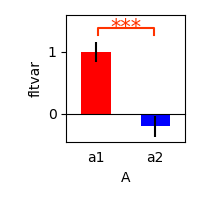•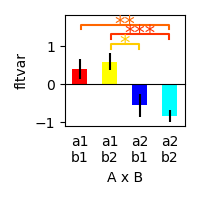## Unambiguous colors

Eelbrain also comes with utilities to quickly generate such color dictionaries. Unambiguous colors are colors for up to 8 categories that are universally distinguishable.

```# add a Factor with more categories
ds['AB'] = ds.eval('A%B').as_factor()

colors = plot.colors_for_oneway(ds['AB'].cells, unambiguous=True)
p = plot.Barplot('fltvar - 0.5', 'AB', data=ds, w=2, xticks=False, colors=colors)
p_colors = plot.ColorList(colors)

colors = plot.colors_for_oneway(ds['AB'].cells, unambiguous=[2, 5, 3, 6])
p = plot.Barplot('fltvar - 0.5', 'AB', data=ds, w=2, xticks=False, colors=colors)
p_colors = plot.ColorList(colors)
```
•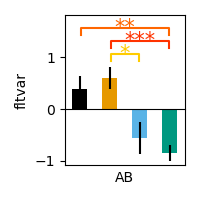•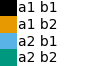•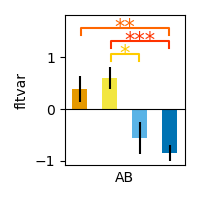•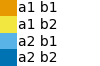## Hue-based colors

Colors can also be generated based on a color wheel.

```cells = 'abc'
colors = plot.colors_for_oneway(cells)
p = plot.ColorList(colors)

cells = 'abc'
colors = plot.colors_for_oneway(cells, hue_start=0.3)
p = plot.ColorList(colors)

cells = 'abcdefgh'
colors = plot.colors_for_oneway(cells)
p = plot.ColorList(colors)
```
•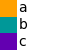•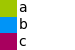•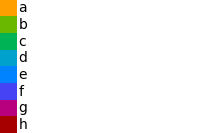Hue and lightness can be used to distinguish two factors in an interaction.

```cells_a = ('a', 'b')
cells_b = ('1', '2')
colors = plot.colors_for_twoway(cells_a, cells_b)
p = plot.ColorGrid(cells_a, cells_b, colors, h=1, w=1)

cells_a = ('a', 'b')
cells_b = ('1', '2', '3')
colors = plot.colors_for_twoway(cells_a, cells_b)
p = plot.ColorGrid(cells_a, cells_b, colors, h=1, w=1)

cells_a = ('a', 'b', 'c')
cells_b = ('1', '2')
colors = plot.colors_for_twoway(cells_a, cells_b, hue_start=0.3)
p = plot.ColorGrid(cells_a, cells_b, colors, h=1, w=1)
```
•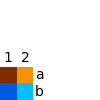•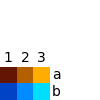•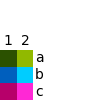Gallery generated by Sphinx-Gallery